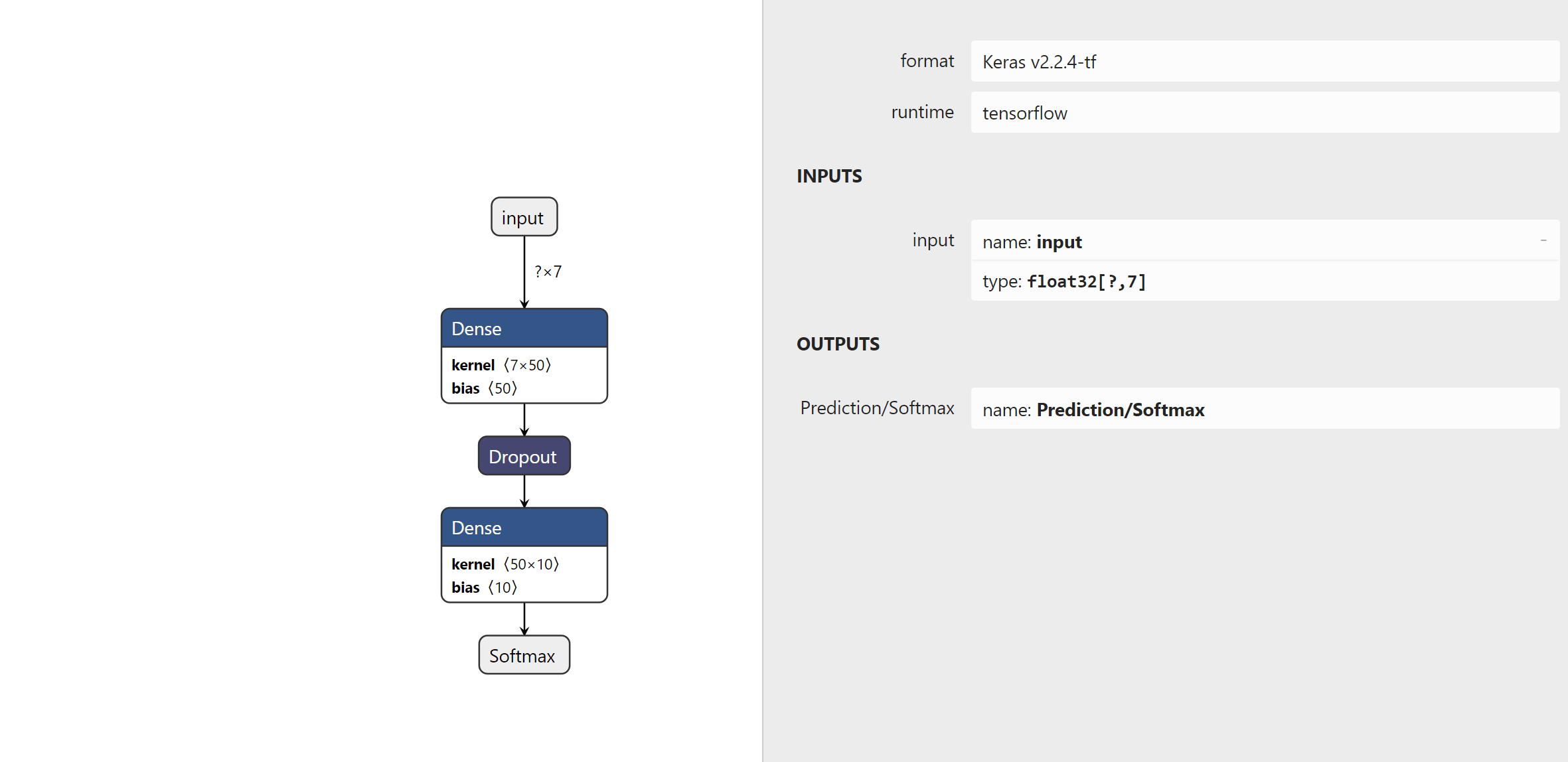# Problem: Export python tensorflow model and load it in ML.Net

I'm trying to play a little bit with ML.Net and keras but i have some questions and problems, maybe i can find the answers:

What i want to achieve: Create a simple NN in Keras, with a hidden layer, 7 inputs and 10 outputs. Export that model, import it in ML.Net and make a prediction there. Part 1: Python code:

``````features_train = ///an array of 3000x7(values between 0 - 1)
labels_train = //an array(vector) of 3000x1 (with the values between 0-9)

model = tf.keras.models.Sequential([
tf.keras.layers.InputLayer(input_shape=(7,), dtype=float, name='Features'),
tf.keras.layers.Dense(50, activation='relu'),
tf.keras.layers.Dropout(0.2),
tf.keras.layers.Dense(10, activation='softmax',name='Prediction/Softmax'),
])

loss='sparse_categorical_crossentropy',
metrics=['accuracy']
)

model.fit(features_train, labels_train, epochs=100)
model.save(path_to_save_file, save_format='tf')
``````

If I save it in h5 and open it with netron, i get the following graph(which looks ok)The first question: If i set the name of the input layer = "Features", why it's not saved in the model as "Features", but as "input"? The output layer is correctly named.

The second question: If i save it as "tf" format, the whole graph get messed in the netron, the names are changed, you can't understand much thing from it(reported also here) Why?

Part 2: I import the model(pb file and variables) in VS, and i put the following code:

``````class House
{
public float Bathrooms { get; set; }
public float SqftLiving { get; set; }
public float SqftLot { get; set; }
public float Floors { get; set; }
public float YearBuild { get; set; }
public float YearRenovated { get; set; }
public float Price { get; set; }

}

class Prediction
{
[VectorType(10)]
public float[] Scores { get; set; }
}

class Program
{
static void Main(string[] args)
{
var mlContext = new MLContext();

var pipeline = mlContext.Transforms.Concatenate("Features",
new[] { "Bathrooms", "SqftLiving", "SqftLot", "Floors", "YearBuild", "YearRenovated", "Price" })
.Append(tensorFlowModel.ScoreTensorFlowModel("Prediction/Softmax", "Features"))
.Append(mlContext.Transforms.CopyColumns("Scores", "Prediction/Softmax"));

var transformer = pipeline.Fit(dataView);

var engine = mlContext.Model.CreatePredictionEngine<House, Prediction>(transformer);

}

}
``````

When i create the pipeline I get this error: Tensorflow.ValueError: 'Could not find operation "Features" inside graph "grap-key-1/".'

The only way i made it was to not use save_model with tf format anymore, but save it as h5 file, then convert it to onnx via keras2onnx and use the onnx file in .net via ApplyOnnxModel.

Using the tf, the input schema looks like this:As you can see, the input is meesed up, the name of the input layer is "serving_default_Features" instead of Features, and you cannot see how the output layer is called.

Do you know why using the pb file generated in python throws that error?

Thanks ^_^